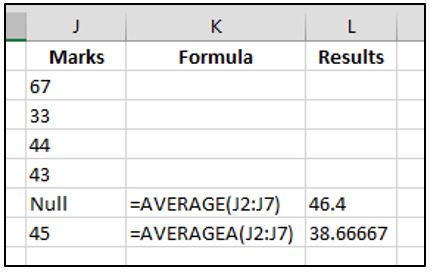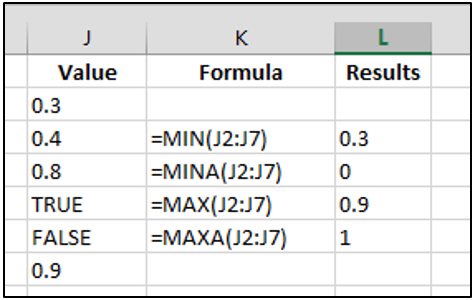Statistical Functions in excel 2013Statistical Functions in excel 2013

To calculate and display the data statistics for lot of amount of data. Excel provides some functions. Following are some important and mostly used of statistical functions:

Average() – This function returns the average of given cell reference or numbers. It can also contains cell name.

Syntax:      =Average(num1, num2, num3,.....)

Example -     =average(5,10,18) Ans. 11

=average(c2,d2,e4,f5)

Averagea() – This function returns the average of given cell references as arguments. If reference has some text, average also count it and returns its average.Syntax:         =Averagea(num1, num2, num3,....)

Example:        =averagea(5,10,18)   Ans. 11

=averagea(c2,d2,e4,f5)

=averagea(a1:c5)

Count() – This function returns the numbers after counting all the cell that contains a numbers, blank cells are not include.

Syntax:         =count(Num1, num2, num2,....)

Example:      =count(5,4,5,3)  Ans. 4

=count(a1:e5, g3:h5)

Counta() – This function returns a number after counting of all the cell that contains any type of values either number or text, blank cells are not included.

Syntax:     =counta(num1, num2, text1,...)

Example:  =counta(5,4,5,3)  Ans. 4

=counta(5,4, “ram”,3,2) Ans. 5

Countblank() – This function returns a number after counting of all the blank cell in the given range as arguments in the function.

Syntax:     =Countblank(cell_range)

Example:    =countblank(a1:c10)

Max()- This function returns a largest value from the given numbers or range as arguments.

Syntax:      =Max(num1, num2,num3,....)

Example:    =max(4,3,9,6)     Ans:  9

Maxa() – This function returns a largest number of given range of arguments. This function included all the logical value in counting, True=1 and false =0.Syntax:     =Maxa(Num1, Num2,Num3,…)

Example:     =maxa(5,6,7,3,8,4)  Ans. 8

Min()- This function returns a smallest value from the given range.

Syntax:  =Min(Num1, Num2,Num3,…)

Example:    =Min(7,6,2,6,9)        Ans: 2

Mina() – This function returns a smallest number of given range of arguments. This function included all the logical value in counting, True=1 and false =0

Syntax:        =Mina(num1, num2, num3,...)

Example:    =mina(4,6,8,3,9) Ans. 3